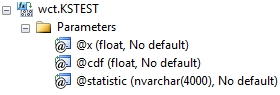# SQL Server ks.test function

KSTEST

Updated: 27 June 2012

Note: This documentation is for the SQL2008 (and later) version of this XLeratorDB function, it is not compatible with SQL Server 2005.

Use the aggregate function KSTEST to compare a sample with a reference probability distribution. The Kolmogorov-Smirnov statistic qualifies a distance between the empirical distribution function of the sample and the cumulative distribution function of the reference sample.
SyntaxArguments
@x
the sample values. @x is an expression of type float or of a type that can be implicitly converted to float.
@cdf
the values of the cumulative distribution function. @cdf is an expression of type float or of a type that can be implicitly converted to float.
@statistic
identifies the return value as either the Kolmogorov-Smirnov statistic (k) or the p-value (p).
Return Types
float
Remarks
·         If @x is null then @x and the corresponding @cdf are not included in the aggregate.
·         If @cdf is null then @cdf and the corresponding @x are not included in the aggregate.
·         @statistic must be invariant for the GROUP.
·         KSTEST is an aggregate function and follows the same conventions as all other aggregate functions in SQL Server.
·         To evaluate two samples, use KSTEST2
Examples
In this example, we compare the sample (-2,-1, 0, 1, 2, 3, 4) to the standard normal distribution.
SELECT wct.KSTEST(x, wct.NORMSDIST(x),'k') as k_observed
,wct.KSTEST(x, wct.NORMSDIST(x),'p') as p_value
FROM (VALUES (-2),(-1),(0),(1),(2),(3),(4)) n(x)
This produces the following result.
k_observed                p_value
---------------------- ----------------------
0.412773317497114      0.135890127950776

In this example, we compare the sample x, consisting of 1,000 randomly generated numbers in the standard normal distribution, to the standard normal distribution.
SELECT wct.KSTEST(x,wct.NORMSDIST(x),'P') as p_value
,wct.KSTEST(x,wct.NORMSDIST(x),'k') as k_observed
FROM (
SELECT k.seriesvalue as x
FROM wctMath.wct.SeriesFloat(0,1,NULL,1000,'N') k
) n(x)

This produces the following result. Your results will be different.
p_value             k_observed
---------------------- ----------------------
0.248195490618504     0.0321196162767327

In this example, we compare the uniformaly random numbers to the gamma distribution with shape 1 and scale 1.
FROM (
SELECT k.seriesvalue as x
FROM wctMath.wct.SeriesFloat(0,1,NULL,100,'R') k
) n(x)
This produces the following result. Your results will be different.
p_value             k_observed
---------------------- ----------------------
1.09546692948201E-12      0.368492604785191

### SupportCopyright 2008-2021 Westclintech LLC         Privacy Policy        Terms of Service